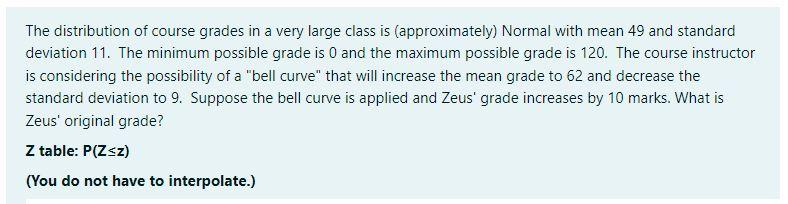# Question The distribution of course grades in a very large class is (approximately) Normal with mean 49 and standard deviation 11. The minimum possible grade is 0 and the maximum possible grade is 120 . The course instructor is considering the possibility of a "bell curve" that will increase the mean grade to 62 and decrease the standard deviation to 9 . Suppose the bell curve is applied and Zeus' grade increases by 10 marks. What is Zeus' original grade? $$Z$$ table: $$P(Z \leq z)$$ (You do not have to interpolate.) The distribution of course grades in a very large class is (approximately) Normal with mean 49 and standard deviation 11. The minimum possible grade is 0 and the maximum possible grade is 120 . The course instructor is considering the possibility of a "bell curve" that will increase the mean grade to 62 and decrease the standard deviation to 9 . Suppose the bell curve is applied and Zeus' grade increases by 10 marks. What is Zeus' original grade? $$Z$$ table: $$P(Z \leq z)$$ (You do not have to interpolate.)AXJJF2 The Asker · Probability and StatisticsTranscribed Image Text: The distribution of course grades in a very large class is (approximately) Normal with mean 49 and standard deviation 11. The minimum possible grade is 0 and the maximum possible grade is 120 . The course instructor is considering the possibility of a "bell curve" that will increase the mean grade to 62 and decrease the standard deviation to 9 . Suppose the bell curve is applied and Zeus' grade increases by 10 marks. What is Zeus' original grade? $$Z$$ table: $$P(Z \leq z)$$ (You do not have to interpolate.) The distribution of course grades in a very large class is (approximately) Normal with mean 49 and standard deviation 11. The minimum possible grade is 0 and the maximum possible grade is 120 . The course instructor is considering the possibility of a "bell curve" that will increase the mean grade to 62 and decrease the standard deviation to 9 . Suppose the bell curve is applied and Zeus' grade increases by 10 marks. What is Zeus' original grade? $$Z$$ table: $$P(Z \leq z)$$ (You do not have to interpolate.)
More
Transcribed Image Text: The distribution of course grades in a very large class is (approximately) Normal with mean 49 and standard deviation 11. The minimum possible grade is 0 and the maximum possible grade is 120 . The course instructor is considering the possibility of a "bell curve" that will increase the mean grade to 62 and decrease the standard deviation to 9 . Suppose the bell curve is applied and Zeus' grade increases by 10 marks. What is Zeus' original grade? $$Z$$ table: $$P(Z \leq z)$$ (You do not have to interpolate.) The distribution of course grades in a very large class is (approximately) Normal with mean 49 and standard deviation 11. The minimum possible grade is 0 and the maximum possible grade is 120 . The course instructor is considering the possibility of a "bell curve" that will increase the mean grade to 62 and decrease the standard deviation to 9 . Suppose the bell curve is applied and Zeus' grade increases by 10 marks. What is Zeus' original grade? $$Z$$ table: $$P(Z \leq z)$$ (You do not have to interpolate.)&#12304;General guidance&#12305;The answer provided below has been developed in a clear step by step manner.Step1/3We can use the formula for z-score to solve this problem:$$\mathrm{{z}=\frac{{{X}-μ}}{σ}}$$where X is the original grade of Zeus, &#956; is the original mean, &#963; is the original standard deviation, and z is the corresponding z-score.Explanation:Please refer to the steps for a detailed explanationExplanation:Please refer to solution in this step.Step2/3We want to find X given that the new mean is 62, the new standard deviation is 9, and Zeus' new grade is 10 marks higher than his original grade. So we have:$$\mathrm{{X}+{10}={62}+{9}\times{z}}$$Solving for z, we get:\( \mathrm{{z}=\frac{{{X}+{10 ... See the full answer